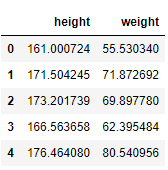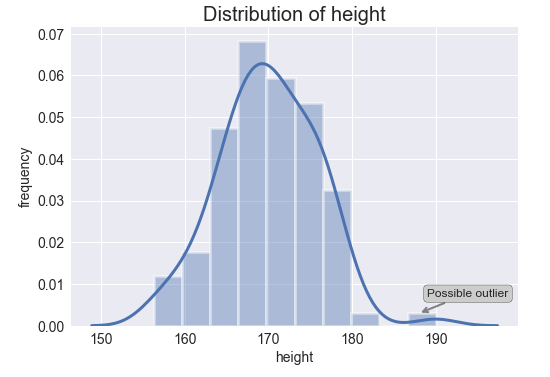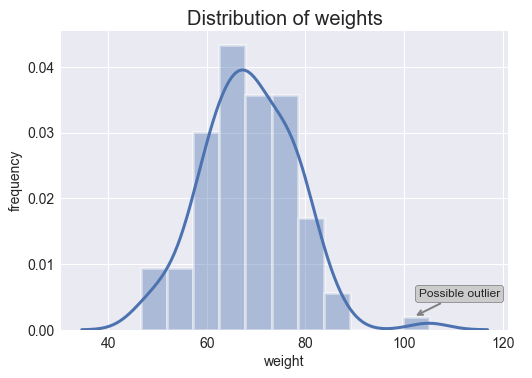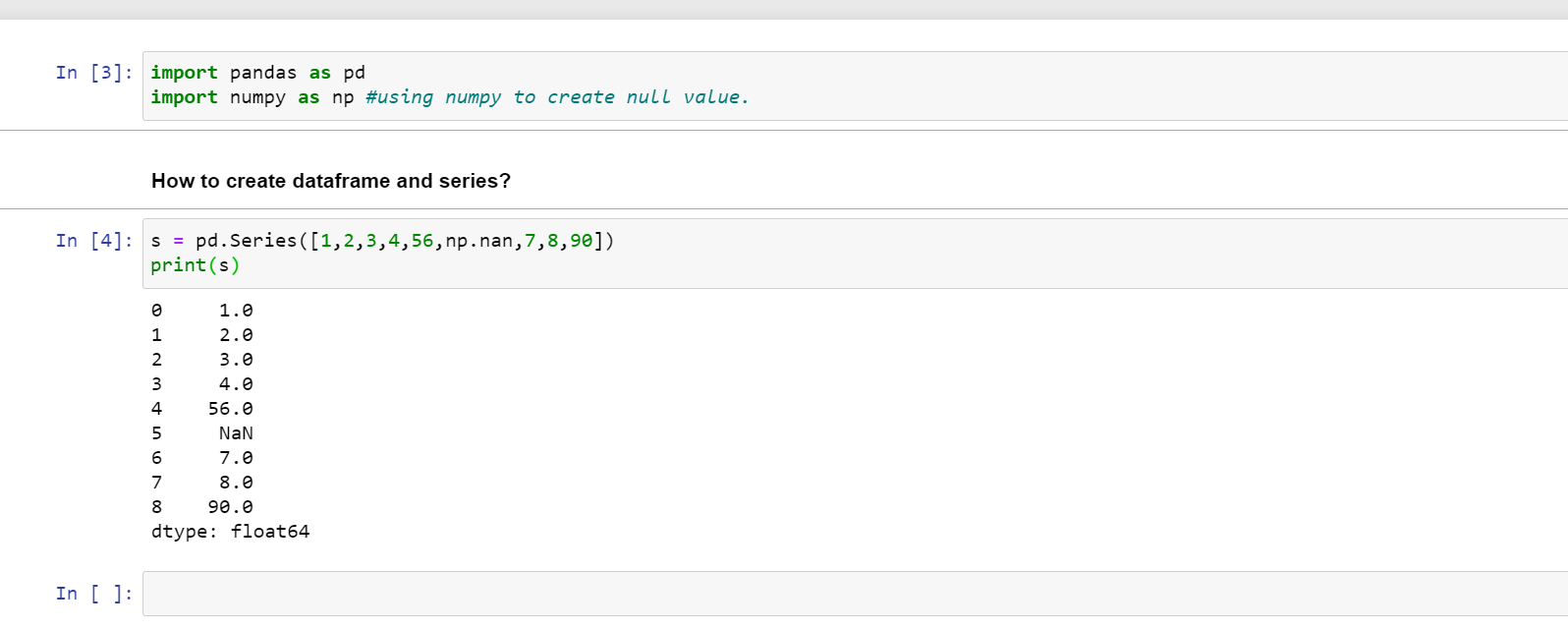1596695280

# Identify Outliers With Pandas, Statsmodels, and Seaborn

#### The complete guide to clean data sets — Part 2Photo by Ine Carriquiry on Unsplash

The success of a machine learning algorithm highly depends on the quality of the data fed into the model. Real-world data is often dirty containing outliers, missing values, wrong data types, irrelevant features, or non-standardized data. The presence of any of these will prevent the machine learning model to properly learn. For this reason, transforming raw data into a useful format is an essential stage in the machine learning process.

**Outliers **are objects in the data set that exhibit some abnormality and deviate significantly from the normal data. In some cases, outliers can provide useful information (e.g. in fraud detection). However, in other cases, they do not provide any helpful knowledge and highly affect the performance of the learning algorithm.

In this article, we will learn how to identify outliers from a data set using multiple techniques such as boxplotsscatterplots, and residuals.

Now, let’s get started 💚

#### Data Set

The data set used for this article contains the weight (kg) and height (cm) of 100 women. As the first step, we load the CSV file into a Pandas data frame using the pandas.read_csv function. Then, we visualize the first 5 rows using the pandas.DataFrame.headmethod.

``````import pandas as pd
import numpy as np
import seaborn as sns
import matplotlib.pyplot as plt
plt.style.use('seaborn')

# visualize the first 5 rows
``````As you may notice, the data set used for this article is really simple (100 observations and 2 features). In real-world problems, you will deal with much more complex data sets. However, the procedures to identify outliers remain the same 💜.

#### Identify outliers

There are many visual and statistical methods to detect outliers. In this post, we will explain in detail 5 tools for identifying outliers in your data set: (1) histograms, (2) box plots, (3) scatter plots, (4) residual values, and (5) Cook’s distance.

#### Histograms

A** histogram** is a common plot to visualize the distribution of a numerical variable. In a histogram, the data is split into intervals also called bins. Each bar’s height represents the frequency of data points within each bin.

The** histograms** for both variables are shown below. The bars are displayed in the shape of a bell-shape curve which indicates that both features (weight and height) are normally distributed. Additionally, the Gaussian kernel density estimation function is depicted as well. This function is an approximation of the probability density function and represents the probability of a continuous variable to fall within a particular range of values.

``````# histogram and kernel density estimation function of the variable heightax = sns.distplot(df_weight.height, hist=True, hist_kws={"edgecolor": 'w', "linewidth": 3}, kde_kws={"linewidth": 3}) # notation indicating a possible outlierax.annotate('Possible outlier', xy=(188,0.0030), xytext=(189,0.0070), fontsize=12, arrowprops=dict(arrowstyle='->', ec='grey', lw=2), bbox = dict(boxstyle="round", fc="0.8")) # ticks plt.xticks(fontsize=14)plt.yticks(fontsize=14) # labels and titleplt.xlabel('height', fontsize=14)plt.ylabel('frequency', fontsize=14)plt.title('Distribution of height', fontsize=20);
````````````# histogram and kernel density estimation function of the variable weightax = sns.distplot(df_weight.weight, hist=True, hist_kws={"edgecolor": 'w', "linewidth": 3}, kde_kws={"linewidth": 3}) # notation indicating a possible outlierax.annotate('Possible outlier', xy=(102, 0.0020), xytext=(103, 0.0050), fontsize=12, arrowprops=dict(arrowstyle='->', ec='grey', lw=2), bbox=dict(boxstyle="round", fc="0.8")) # ticks plt.xticks(fontsize=14)plt.yticks(fontsize=14) # labels and titleplt.xlabel('weight', fontsize=14)plt.ylabel('frequency', fontsize=14)plt.title('Distribution of weights', fontsize=20);
``````As you can see above, it seems that both variables present an outlier (isolated bar). It is important to bear in mind that histograms do not identify outliers statistically as box plots do. On the contrary, the identification of outliers with histograms is entirely visual and depends on our personal view.

#pandas #statistics #python #programming #data-science

## Buddha Community1586702221

## Python Pandas Objects - Pandas Series and Pandas Dataframe

In this post, we will learn about pandas’ data structures/objects. Pandas provide two type of data structures:-

### Pandas Series

Pandas Series is a one dimensional indexed data, which can hold datatypes like integer, string, boolean, float, python object etc. A Pandas Series can hold only one data type at a time. The axis label of the data is called the index of the series. The labels need not to be unique but must be a hashable type. The index of the series can be integer, string and even time-series data. In general, Pandas Series is nothing but a column of an excel sheet with row index being the index of the series.

### Pandas Dataframe

Pandas dataframe is a primary data structure of pandas. Pandas dataframe is a two-dimensional size mutable array with both flexible row indices and flexible column names. In general, it is just like an excel sheet or SQL table. It can also be seen as a python’s dict-like container for series objects.

#python #python-pandas #pandas-dataframe #pandas-series #pandas-tutorial1602550800

## Pandas in Python

Pandas is used for data manipulation, analysis and cleaning.

What are Data Frames and Series?

Dataframe is a two dimensional, size mutable, potentially heterogeneous tabular data.

It contains rows and columns, arithmetic operations can be applied on both rows and columns.

Series is a one dimensional label array capable of holding data of any type. It can be integer, float, string, python objects etc. Panda series is nothing but a column in an excel sheet.

### How to create dataframe and series?

s = pd.Series([1,2,3,4,56,np.nan,7,8,90])

print(s)How to create a dataframe by passing a numpy array?

1. d= pd.date_range(‘20200809’,periods=15)
2. print(d)
3. df = pd.DataFrame(np.random.randn(15,4), index= d, columns = [‘A’,’B’,’C’,’D’])
4. print(df)

#pandas-series #pandas #pandas-in-python #pandas-dataframe #python1596695280

## Identify Outliers With Pandas, Statsmodels, and Seaborn

#### The complete guide to clean data sets — Part 2Photo by Ine Carriquiry on Unsplash

The success of a machine learning algorithm highly depends on the quality of the data fed into the model. Real-world data is often dirty containing outliers, missing values, wrong data types, irrelevant features, or non-standardized data. The presence of any of these will prevent the machine learning model to properly learn. For this reason, transforming raw data into a useful format is an essential stage in the machine learning process.

**Outliers **are objects in the data set that exhibit some abnormality and deviate significantly from the normal data. In some cases, outliers can provide useful information (e.g. in fraud detection). However, in other cases, they do not provide any helpful knowledge and highly affect the performance of the learning algorithm.

In this article, we will learn how to identify outliers from a data set using multiple techniques such as boxplotsscatterplots, and residuals.

Now, let’s get started 💚

#### Data Set

The data set used for this article contains the weight (kg) and height (cm) of 100 women. As the first step, we load the CSV file into a Pandas data frame using the pandas.read_csv function. Then, we visualize the first 5 rows using the pandas.DataFrame.headmethod.

``````import pandas as pd
import numpy as np
import seaborn as sns
import matplotlib.pyplot as plt
plt.style.use('seaborn')

# visualize the first 5 rows
``````As you may notice, the data set used for this article is really simple (100 observations and 2 features). In real-world problems, you will deal with much more complex data sets. However, the procedures to identify outliers remain the same 💜.

#### Identify outliers

There are many visual and statistical methods to detect outliers. In this post, we will explain in detail 5 tools for identifying outliers in your data set: (1) histograms, (2) box plots, (3) scatter plots, (4) residual values, and (5) Cook’s distance.

#### Histograms

A** histogram** is a common plot to visualize the distribution of a numerical variable. In a histogram, the data is split into intervals also called bins. Each bar’s height represents the frequency of data points within each bin.

The** histograms** for both variables are shown below. The bars are displayed in the shape of a bell-shape curve which indicates that both features (weight and height) are normally distributed. Additionally, the Gaussian kernel density estimation function is depicted as well. This function is an approximation of the probability density function and represents the probability of a continuous variable to fall within a particular range of values.

``````# histogram and kernel density estimation function of the variable heightax = sns.distplot(df_weight.height, hist=True, hist_kws={"edgecolor": 'w', "linewidth": 3}, kde_kws={"linewidth": 3}) # notation indicating a possible outlierax.annotate('Possible outlier', xy=(188,0.0030), xytext=(189,0.0070), fontsize=12, arrowprops=dict(arrowstyle='->', ec='grey', lw=2), bbox = dict(boxstyle="round", fc="0.8")) # ticks plt.xticks(fontsize=14)plt.yticks(fontsize=14) # labels and titleplt.xlabel('height', fontsize=14)plt.ylabel('frequency', fontsize=14)plt.title('Distribution of height', fontsize=20);
````````````# histogram and kernel density estimation function of the variable weightax = sns.distplot(df_weight.weight, hist=True, hist_kws={"edgecolor": 'w', "linewidth": 3}, kde_kws={"linewidth": 3}) # notation indicating a possible outlierax.annotate('Possible outlier', xy=(102, 0.0020), xytext=(103, 0.0050), fontsize=12, arrowprops=dict(arrowstyle='->', ec='grey', lw=2), bbox=dict(boxstyle="round", fc="0.8")) # ticks plt.xticks(fontsize=14)plt.yticks(fontsize=14) # labels and titleplt.xlabel('weight', fontsize=14)plt.ylabel('frequency', fontsize=14)plt.title('Distribution of weights', fontsize=20);
``````As you can see above, it seems that both variables present an outlier (isolated bar). It is important to bear in mind that histograms do not identify outliers statistically as box plots do. On the contrary, the identification of outliers with histograms is entirely visual and depends on our personal view.

#pandas #statistics #python #programming #data-science1616050935

## WORKING WITH GROUPBY IN PANDAS

In my last post, I mentioned the groupby technique  in Pandas library. After creating a groupby object, it is limited to make calculations on grouped data using groupby’s own functions. For example, in the last lesson, we were able to use a few functions such as mean or sum on the object we created with groupby. But with the aggregate () method, we can use both the functions we have written and the methods used with groupby. I will show how to work with groupby in this post.

#pandas-groupby #python-pandas #pandas #data-preprocessing #pandas-tutorial1616395265

## Reading and Writing Data in Pandas

In my last post, I mentioned summarizing and computing descriptive statistics  using the Pandas library. To work with data in Pandas, it is necessary to load the data set first. Reading the data set is one of the important stages of data analysis. In this post, I will talk about reading and writing data.

Before starting the topic, our Medium page includes posts on data science, artificial intelligence, machine learning, and deep learning. Please don’t forget to follow us on Medium 🌱 to see these posts and the latest posts.

Let’s get started.